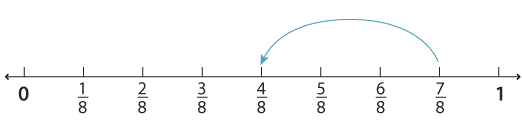Subtraction of fractions with the same denominator

Subtraction of two fractions with the same denominator can be thought of as steps on the number line.

To subtract $$\dfrac{3}{8} \text{from} \dfrac{7}{8}$$ we start at $$\dfrac{7}{8}$$ and take a step of $$\dfrac{3}{8} \text{to get} \dfrac{4}{8}$$.Detailed description

We write $$\dfrac{7}{8} − \dfrac{3}{8} = \dfrac{4}{8}$$.